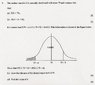# Statistics Question - Normal Distribution

• Chewy0087

#### Chewy0087

Statistics Question - Normal Distribution SOLVED

## Homework Statement

Hey, the question is shown in the attached document.

## Homework Equations

Mean = 79 Standard Deviation = 12

## The Attempt at a Solution

I dispise these questions, and I'm failing to grasp them at all.

I've done the first two parts fine, but it's part c I'm struggling on.

I've tried using the z function getting me to;

P (Z $$\leq$$ $$\frac{b}{12}$$ ) - P ( Z $$\leq$$ $$\frac{-a}{12}$$ ) = 0.375

But I've got no idea what I'm doing :/ this question has totally thrown me, all of the methods I've used replacing the 2nd equation into the first have given me useless answers, I can usually do these questions fine, but I'm struggling here, any help would be great!

#### Attachments

•stats.jpg
41.9 KB · Views: 332
Last edited: